# Behind The Code

Thanks so much for checking out Platformer Toolkit. I hope the game gave you an insight into how small changes, adjustments, and design decisions can radically change how a game feels to play.

If you’re interested in taking the next step and actually making a platformer for yourself, you might be interested in how some of the code works. So I've attached the scripts that control Kit's movements - you can find them attached below.

In this blog I’m going to walk you through the code blocks that drive some of Kit’s core features, like acceleration, the jump arc, coyote time, and variable jump height.

This is an intermediate level blog, so if you wish to apply these yourself you’ll need some familiarity with a game engine, and some programming skills to make the code all snap together properly. If you get really stuck, drop a comment below and I or someone else will help out.

### Running with acceleration, deceleration, turn speed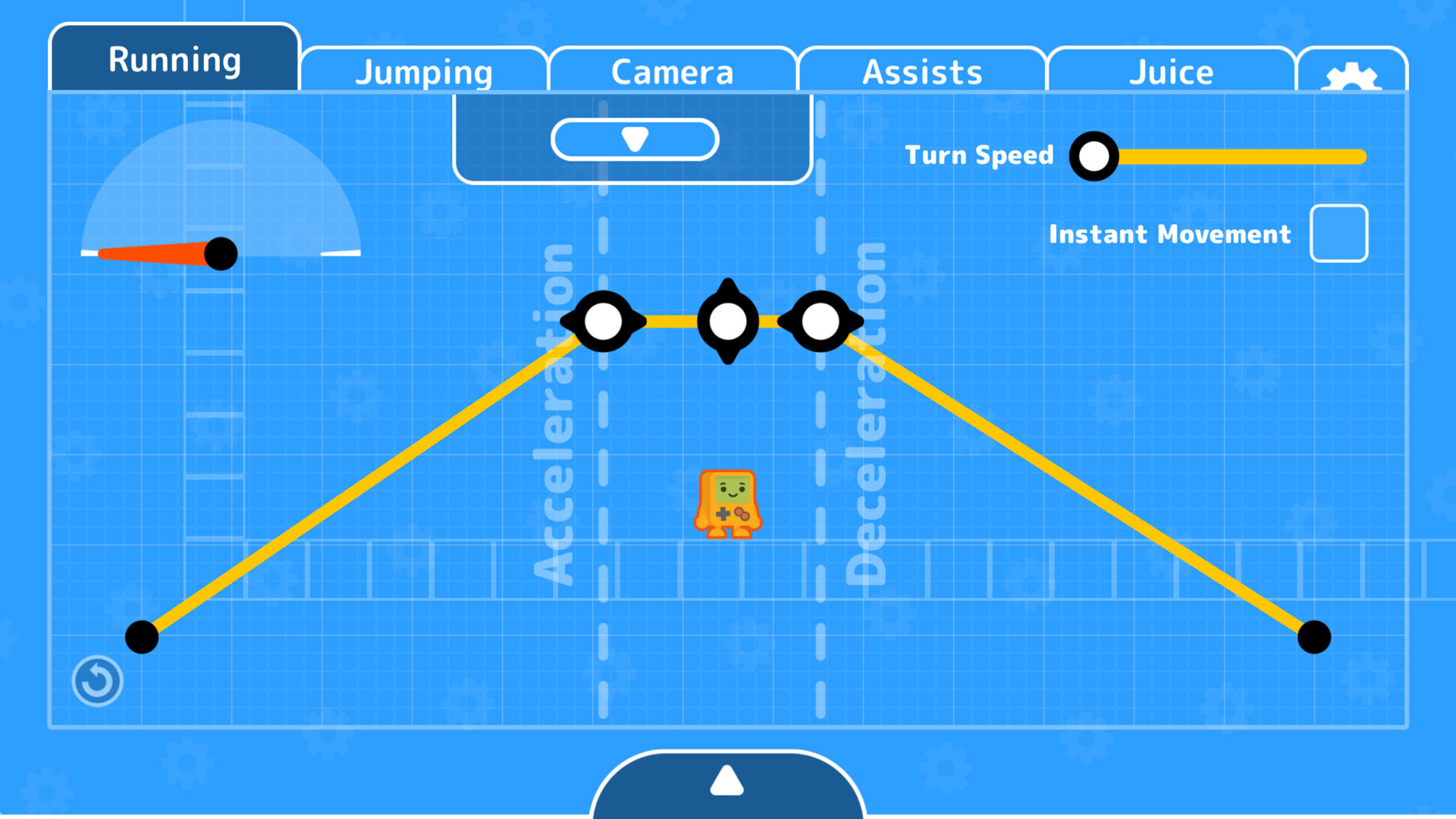On the running page of the toolkit we not only get to change how fast Kit moves, but also how fast she accelerates, decelerates, and turns. Here’s how that is achieved.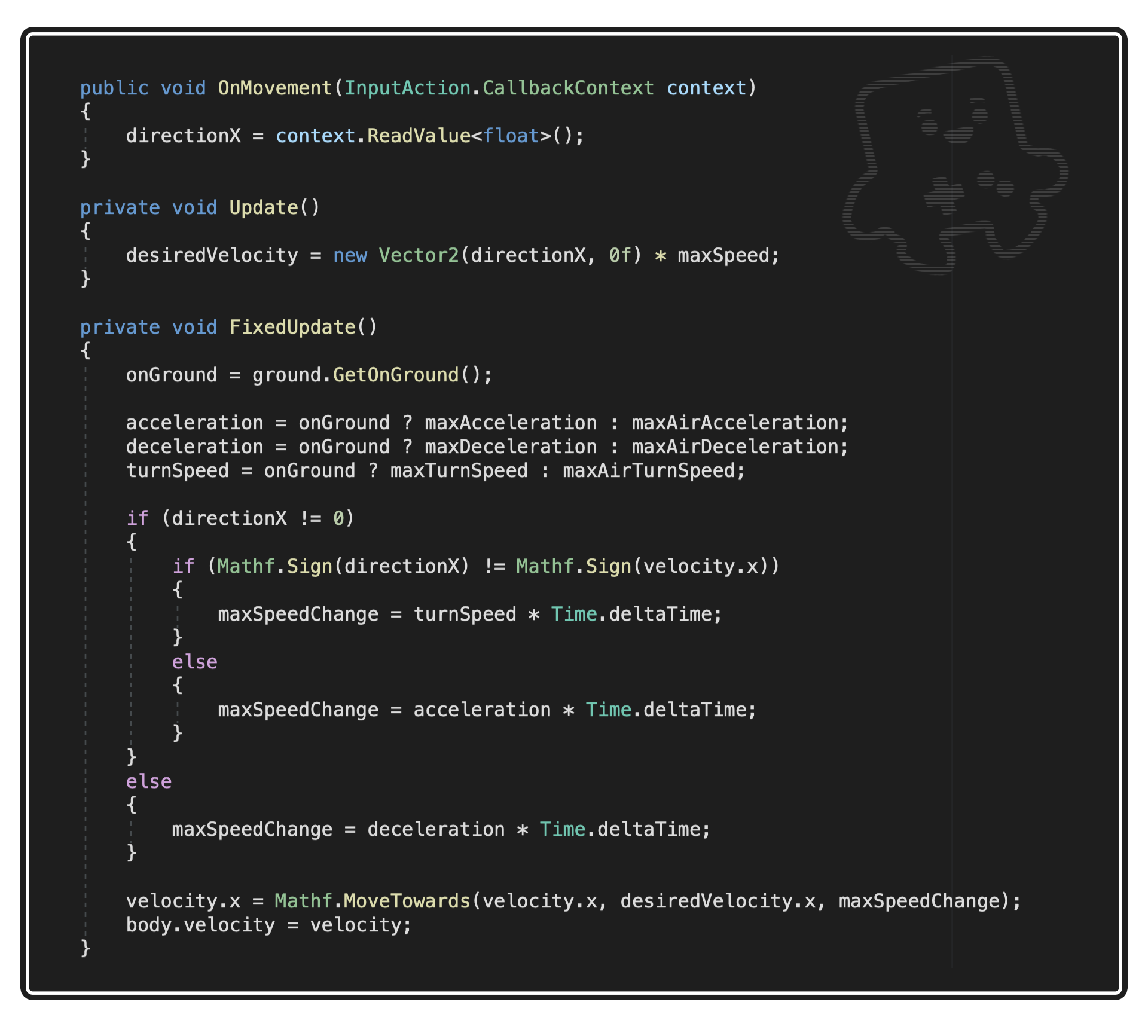```directionX = context.ReadValue<float>();
```

So...we find out which direction the player is inputting, and store it in directionX. It’s a float which is either -1 (left), 0 (no input), or 1 (right).

```desiredVelocity = new Vector2(directionX, 0f) * maxSpeed;
```

We can then get the desired velocity, which is the player’s direction multiplied by Kit’s max speed.

```onGround = ground.GetOnGround();
acceleration = onGround ? maxAcceleration : maxAirAcceleration;
```

In FixedUpdate, we first check if the player is on the ground or in mid-air, and put the correct stats into the acceleration, deceleration, and turnSpeed variables.

``` if (directionX != 0)
{
if (Mathf.Sign(directionX) != Mathf.Sign(velocity.x))
{
```

We then check if the player is currently inputting a direction. If they are, we check if the sign (i.e. positive or negative) of the input direction matches the sign of Kit’s current direction - if they don’t, it means Kit is in the process of turning around, so we should use the turn speed. If they’re aligned, we should use acceleration instead. And if no button is being pressed, use deceleration.

```velocity.x = Mathf.MoveTowards(velocity.x, desiredVelocity.x, maxSpeedChange);
body.velocity = velocity;
```

Then, we move from Kit’s current velocity, to her desired velocity, at the rate of whatever we just picked, and apply it to the Rigidbody.

### Creating a jump from three stats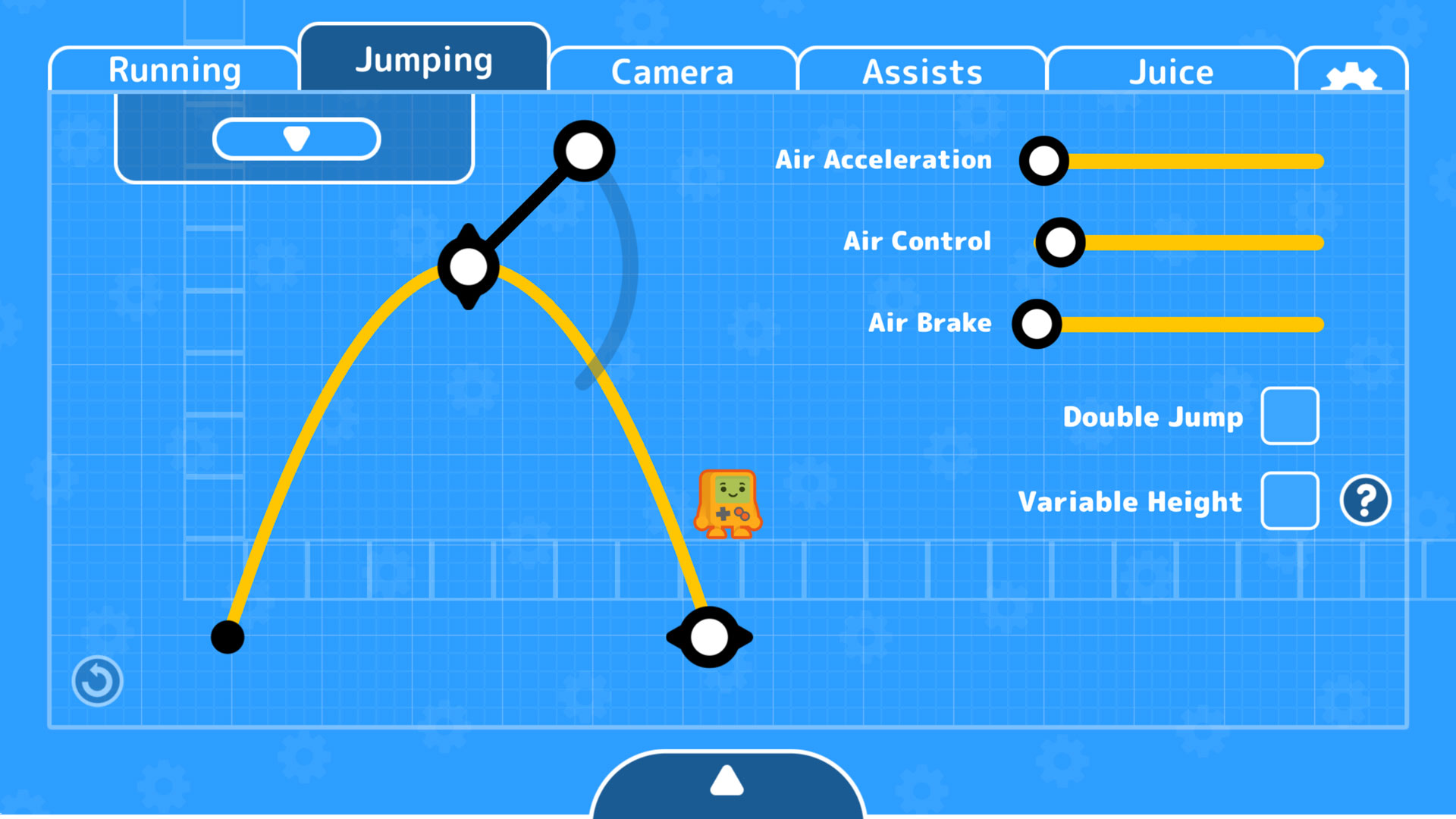The jumping panel allows us to define Kit’s jump by two simple numbers: how high should she jump, and how long should it take to reach that apex before coming back down? And when coming down, we can set a downward gravity multiplier for a snappier landing.

To calculate all this, I use some complex maths that I definitely don’t understand. Thanks to the GMTK Discord for helping with this one!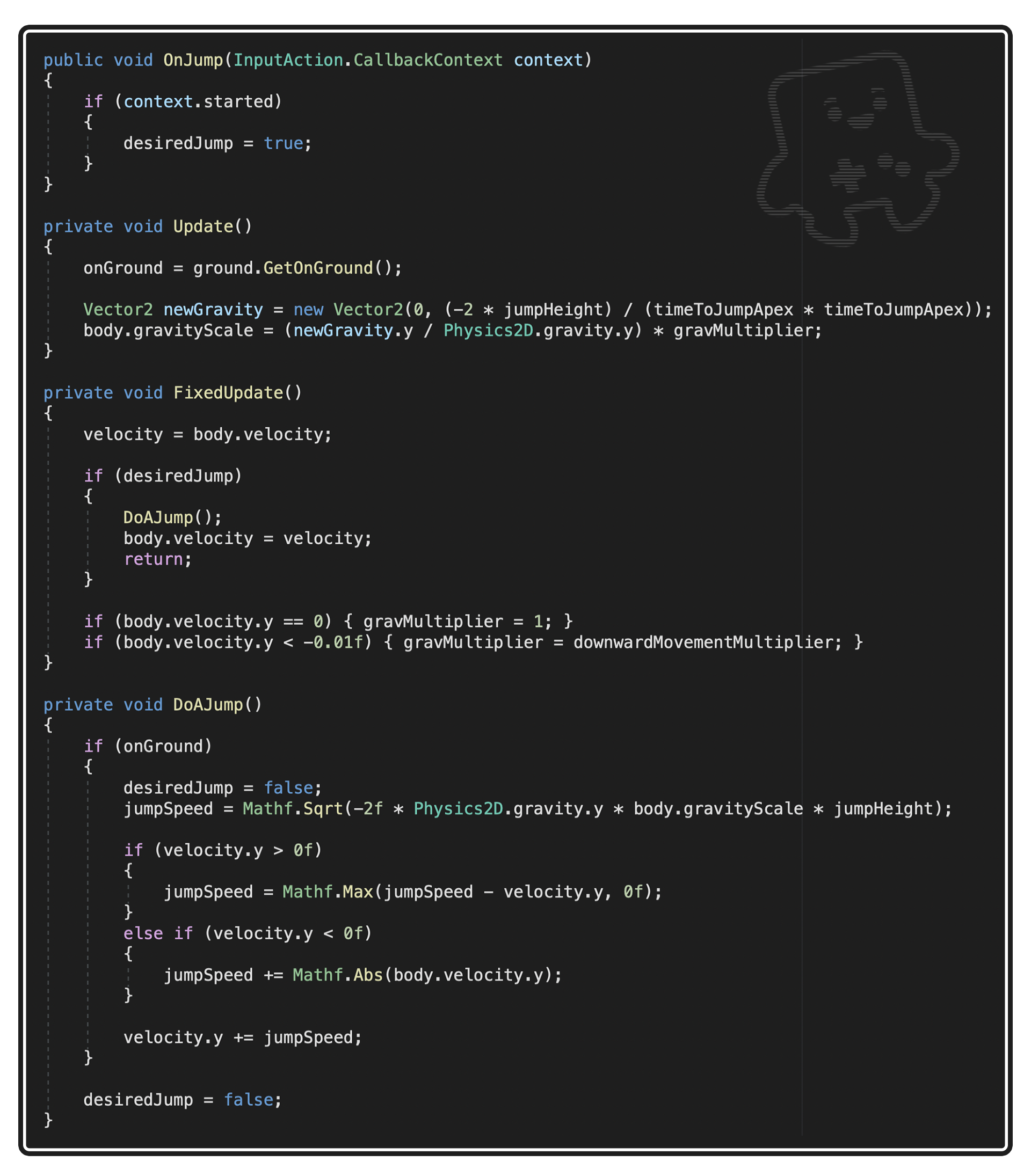We start by reading the input from the jump button, and passing it to FixedUpdate.

```Vector2 newGravity = new Vector2(0, (-2 * jumpHeight) / (timeToJumpApex * timeToJumpApex));
body.gravityScale = (newGravity.y / Physics2D.gravity.y) * gravMultiplier;
```

In Update, we change the character’s gravity scale, using the jump height and duration (this is the maths bit I don't understand). It’s divided by the physics engine’s gravity, and multiplied by a, erm, multiplier.

```if (body.velocity.y == 0) { gravMultiplier = 1; }
if (body.velocity.y < -0.01f) { gravMultiplier = downwardMovementMultiplier; }
```

That multiplier is determined in FixedUpdate: if Kit’s velocity is negative (i.e, she’s falling), we kick on the downward gravity multiplier. Otherwise, it’s a flat one.

```jumpSpeed = Mathf.Sqrt(-2f * Physics2D.gravity.y * body.gravityScale * jumpHeight);
if (velocity.y > 0f)
{
jumpSpeed = Mathf.Max(jumpSpeed - velocity.y, 0f);
}
else if (velocity.y < 0f)
{
jumpSpeed += Mathf.Abs(body.velocity.y);
}
```

Then, when the jump actually happens, we create a jumpSpeed variable using some more complicated maths (help me...) and apply it to the character. By determining our character’s velocity and changing the jumpSpeed to match, we make sure we get the same jump even if we’re currently rising or falling (handy for double jumps and springy pads).

```velocity.y += jumpSpeed;
```

We then apply to the Rigidbody!

Variable jump height means the height of your jump is determined by how long you hold down the button. We've just set the maximum height, so now let’s add a few extra tweaks to make it so the character drops when you let go of the jump button.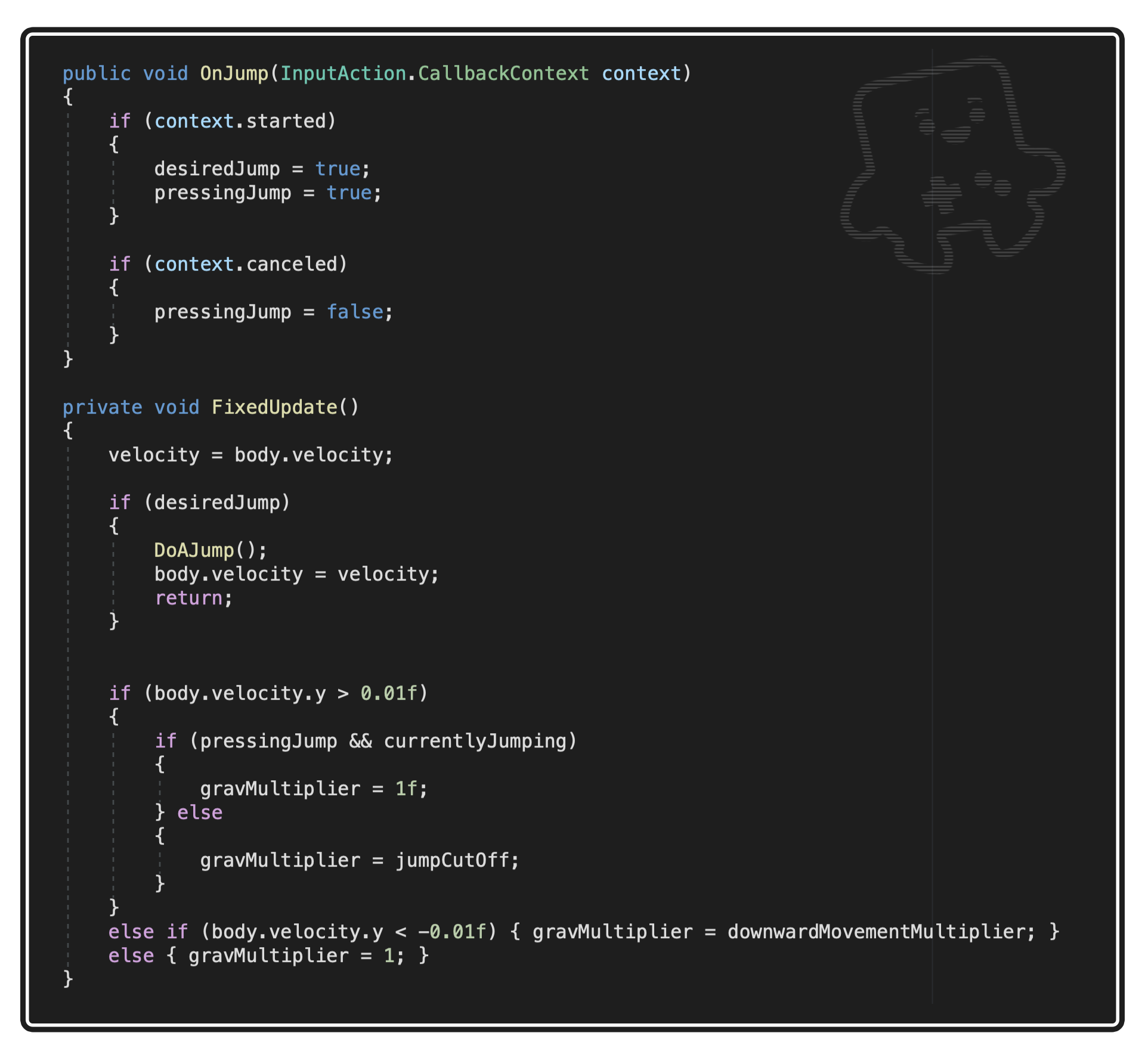First...

```if (context.started)
{
desiredJump = true;
pressingJump = true;
}
if (context.canceled)
{
pressingJump = false;
}
```

we can use Unity’s input system to determined when we start and cancel the input, which we save in the pressingJump bool. (We also need a currentlyJumping bool, which is made true when you jump, and false when you hit the ground - it's not shown in the code block above, but it's in the full script).

```if (body. velocity.y > 0.01f)
{
if (pressingJump && currentlyJumping)
{
gravMultiplier = 1f;
} else
{
gravMultiplier = jumpCutoff;
}
```

Then, if Kit’s velocity is less than 0 (i.e. she’s going up), and pressingJump and currentlyJumping are not both true, it means we’ve let go of the jump button. So apply a gravity multiplier, just like the previous step. This one’s called jumpCutOff.

The assist panel has a few features that allow us to bias the game's controls in the player's favour.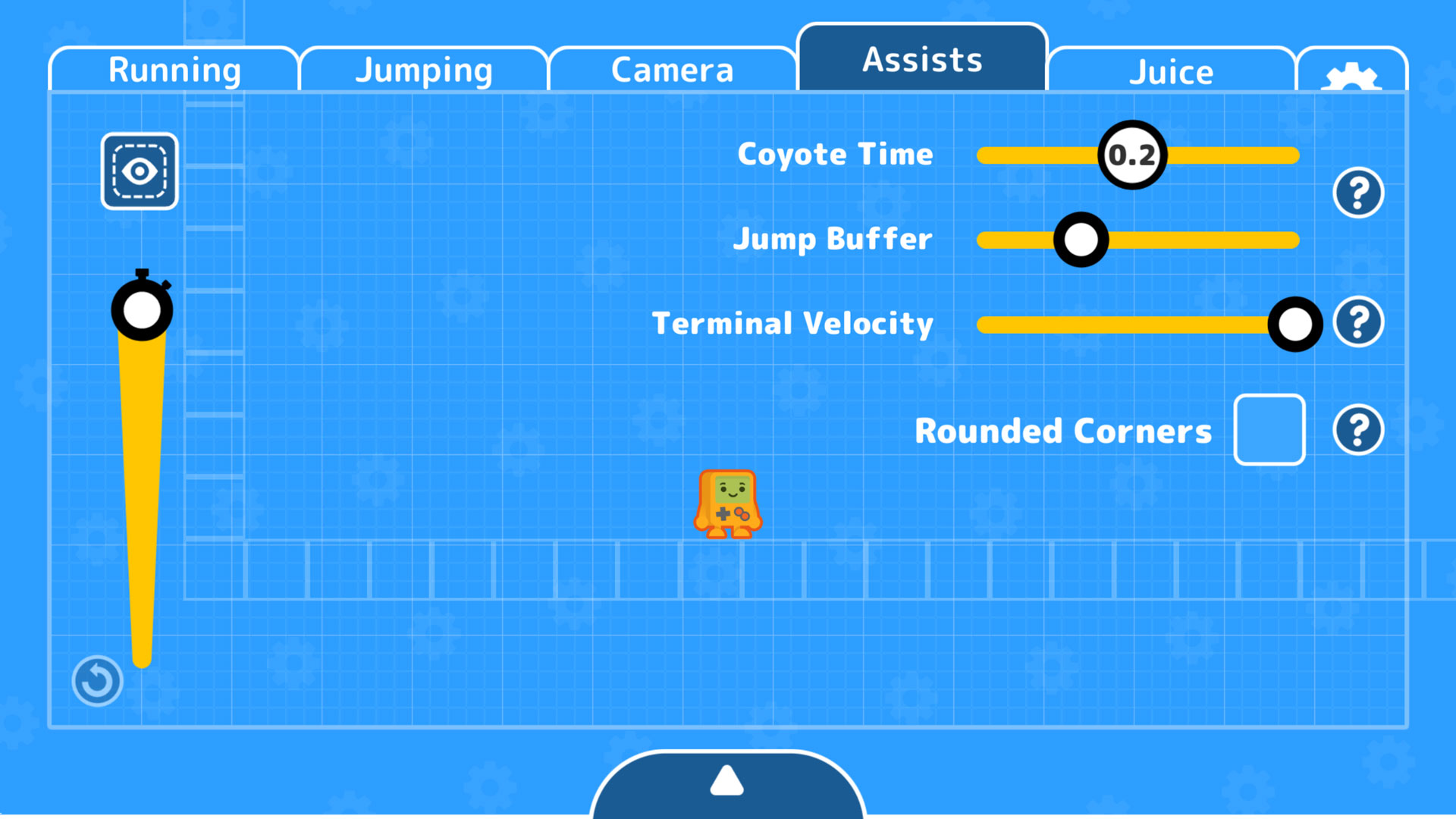Coyote time is one of those handy player grace features. In this one, you can still jump even if you’ve just run off the edge of a platform. Here’s how it works.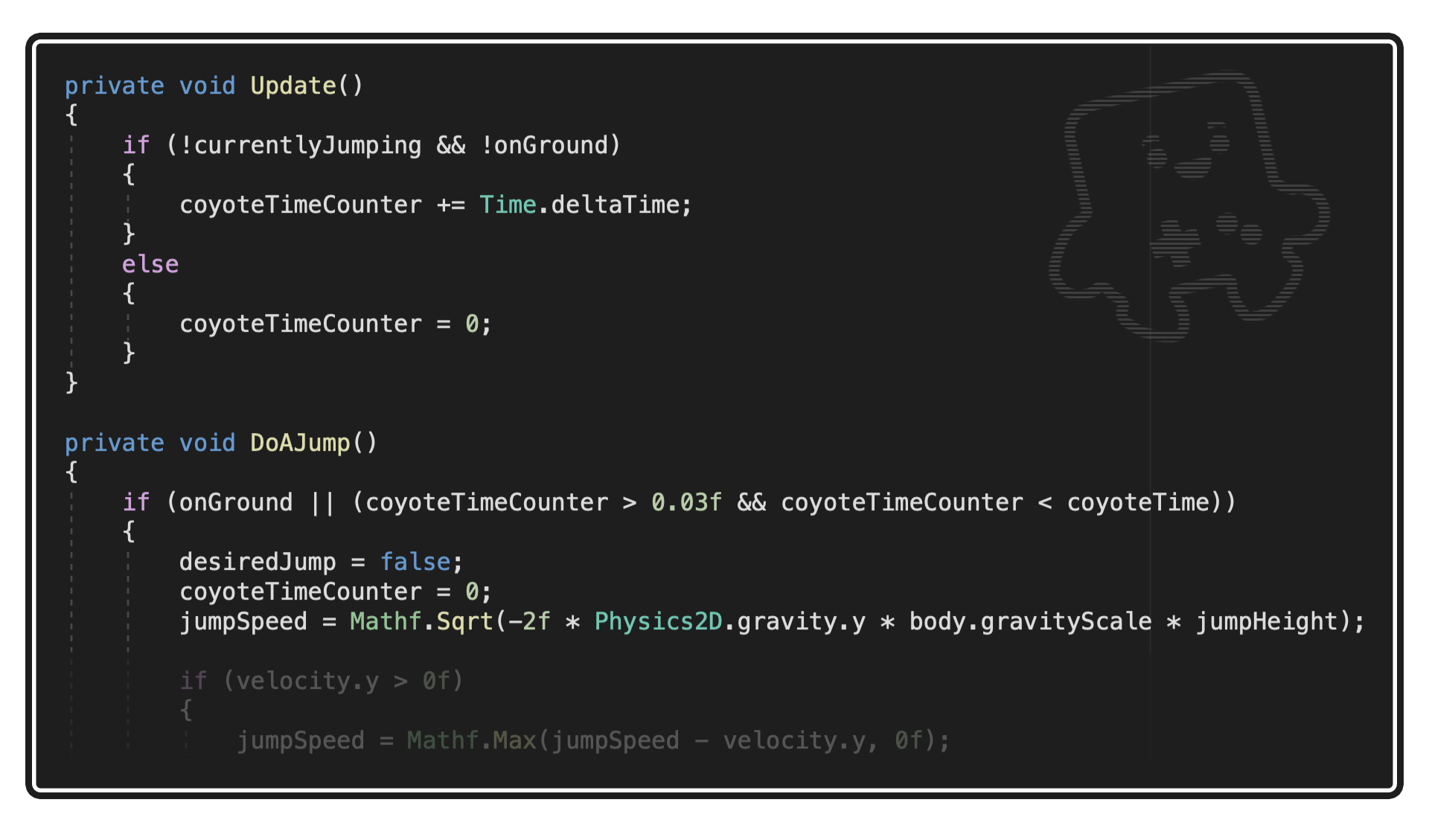So first up...

```if (!currentlyJumping && !onGround)
{
coyoteTimeCounter += Time.deltaTime;
}
else
{
coyoteTimeCounter = 0;
}
```

In update, we check if the player is not on the ground, and also not jumping - this means they’ve walked off the edge of a platform. At this point, we start counting up the Coyote Time counter.

```if (onGround | | (coyoteTimeCounter > 0.03f && coyoteTimeCounter < coyoteTime))
```

Then, we add another check before letting the player do a jump: is the Coyote Time counter below Coyote Time (a number set by the developer - usually something like 0.2). If it is, allow the jump to happen and reset the counter.

Another grace mechanic is jump buffer. This means we can press jump a few frames before hitting the ground, and it will still trigger the jump when we land.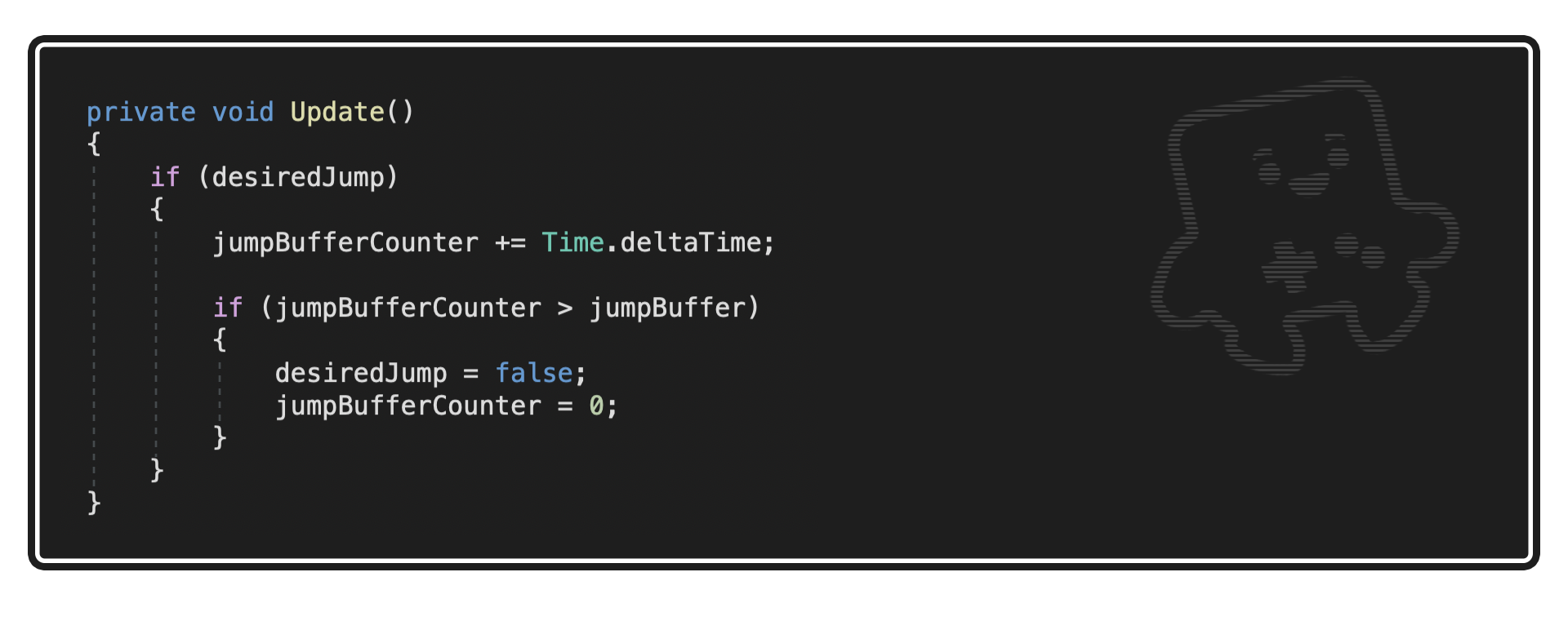So, when we hit the jump button we tell the game we desire a jump, and it checks if we’re on the ground (or in Coyote Time). If we’re not, it immediately turns off the “desiredJump” bool.

```jumpBufferCounter += Time.deltaTime;
if (jumpBufferCounter > jumpBuffer)
{
desiredJump = false;
```

But now, we instead start a jump buffer counter - and only turn off desiredJump if the counter hits its max. This way, the game will repeatedly try to jump for a few frames - and will successfully trigger a jump when Kit hits the ground.

Those are the main ones! I couldn't have done this without some help, so here's where I stole borrowed code from, or received help:

Cheers!

Mark

## Files

Character Controller Unity Scripts 10 kB
7 days ago

## Get Platformer Toolkit

movementLimiter  jumpTester  optionsManagement  labOpener

These four classes seem never defined in the scripts? They are causing errors

this code is one of the best things that happened to me this week... it doesn't compete against much either, but it's really spectacular
I download the code and i'm triyng to add the characterMovement to my game, but I need help with this line in the code in particular:

[SerializeField] movementLimiter moveLimit; (13 line characterMovement)

Unity says that the movementLimiter namespace doesn't exist. I need download some package, or i need to add other script, or how can i fix the error?
Sorry, I'm new to programming

Hello, I'm having the same problem, did you find a solution? thanks

not yet :(

Uh huhm, interesting...

Great work!